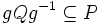# Sylow's theorem

## Contents

This article gives the statement, and possibly proof, of a basic fact in group theory.
View a complete list of basic facts in group theory
VIEW FACTS USING THIS: directly | directly or indirectly, upto two steps | directly or indirectly, upto three steps|
This fact is related to: Sylow theory
View other facts related to Sylow theoryView terms related to Sylow theory |

## Statement

### Verbal statement

The Sylow's theorem(s) give(s) information about the existence of$p$-Sylow subgroups of a finite group, as well as the relation among them. More specifically, given a finite group:

• Existence: For any prime$p$, there exists a$p$-Sylow subgroup
• Conjugacy: Any two$p$-Sylow subgroups are conjugate in the whole group
• Domination: Any$p$-subgroup is contained inside some$p$-Sylow subgroup
• Congruence: The number of$p$-Sylow subgroups divides the index of any$p$-Sylow subgroup and is also congruent to$1$ modulo$p$.

### Statement with symbols

Let$G$ be a finite group and$p$ a prime. A subgroup of$G$ is termed a$p$-Sylow subgroup if its order is a power of$p$ and its index is relatively prime to$p$. Note that by Lagrange's theorem, the order of a Sylow subgroup is the largest power of$p$ dividing the order of$G$, and thus, it is a multiple of the order of any$p$-subgroup (subgroup whose order is a power of$p$) of$G$.

Then Sylow's theorem states that:

1. Existence: There exists a$p$-Sylow subgroup$P$ of$G$
2. Conjugacy: If$P$ and$Q$ are$p$-Sylow subgroups of$G$ then there exists$g$ in$G$ such that$gPg^{-1} = Q$ (i.e.,$P$ and$Q$ are conjugate subgroups)
3. Domination: Let$P$ be a$p$-Sylow subgroup and$Q$ a$p$-group. Then there exists a$g$ in$G$ such that$gQg^{-1} \subseteq P$.
4. Congruence: Let$Syl_p(G)$ denote the set of$p$-Sylow subgroups of$G$ and$n_p$ denote the cardinality of$Syl_p(G)$. Then,$n_p \equiv 1\mod p$.

## Related facts

### Corollaries

There are other corollaries too, many of which factor through these corollaries.

## Proof breakup

1. Existence: For full proof, refer: Sylow subgroups exist
2. Conjugacy: This follows from Domination, though it can also be proved through the methods used to establish Congruence. For full proof, refer: Sylow implies order-conjugate
3. Domination: For full proof, refer: Sylow implies order-dominating
4. Congruence: For full proof, refer: Congruence condition on Sylow numbers